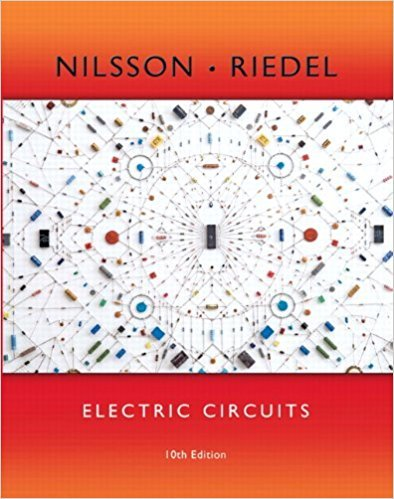×
×

# The switch in the circuit seen in Fig. P7.10 has been in position 1 for a long time. AtISBN: 9780133760033 390

## Solution for problem 7.10 Chapter 7

Electric Circuits | 10th Edition

• Textbook Solutions
• 2901 Step-by-step solutions solved by professors and subject experts
• Get 24/7 help from StudySoup virtual teaching assistantsElectric Circuits | 10th Edition

4 5 1 359 Reviews
31
1
Problem 7.10

The switch in the circuit seen in Fig. P7.10 has been in position 1 for a long time. At the switch moves instantaneously to position 2. Find the value of R so that of the initial energy stored in the 10 mH inductor is dissipated in R in Figure P7.10 100 R t 0 10 mH 1 2 5 A 10 ms.

Step-by-Step Solution:
Step 1 of 3

Introduction (Part 1) What is mechanics  The science that describes and predicts the conditions of rest or motion of bodies under the action of forces.  What happens to this body o Does it move (Dynamic) o Does it stay still (Static) o Does it deform/ deflect Mechanics is Comprised of Multiple Topics  Rigid bodies o...

Step 2 of 3

Step 3 of 3

##### ISBN: 9780133760033

This full solution covers the following key subjects: . This expansive textbook survival guide covers 107 chapters, and 1357 solutions. This textbook survival guide was created for the textbook: Electric Circuits, edition: 10. The answer to “The switch in the circuit seen in Fig. P7.10 has been in position 1 for a long time. At the switch moves instantaneously to position 2. Find the value of R so that of the initial energy stored in the 10 mH inductor is dissipated in R in Figure P7.10 100 R t 0 10 mH 1 2 5 A 10 ms.” is broken down into a number of easy to follow steps, and 62 words. Since the solution to 7.10 from 7 chapter was answered, more than 215 students have viewed the full step-by-step answer. The full step-by-step solution to problem: 7.10 from chapter: 7 was answered by , our top Engineering and Tech solution expert on 03/13/18, 07:48PM. Electric Circuits was written by and is associated to the ISBN: 9780133760033.

Unlock Textbook Solution